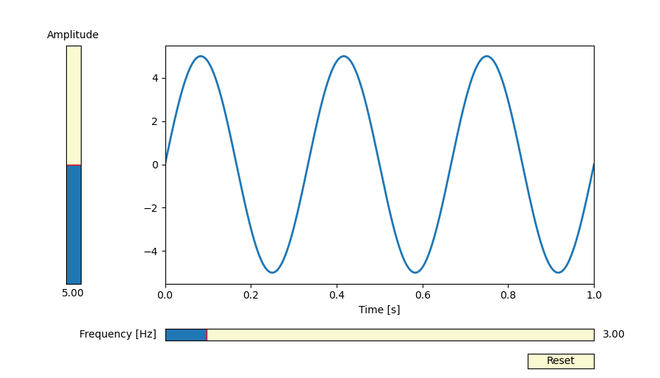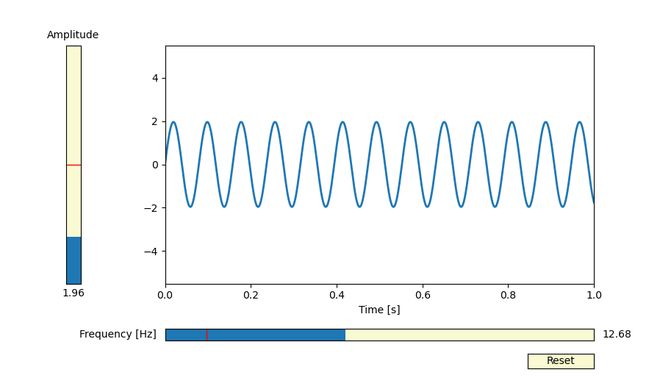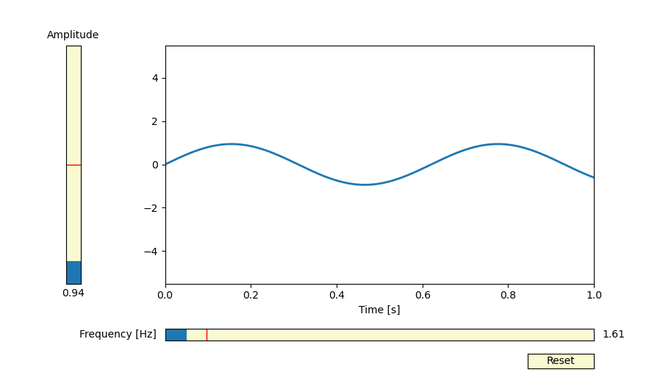Add a vertical slider with matplotlib

• Last Updated : 17 May, 2021

Matplotlib not only allows static graphs, but we can also prepare plots that can be modified interactively.  For this, we can use the Sliders widget present in the widgets submodule to control the visual properties of your plot.

The only difference between horizontal and vertical Sliders being the presence of an additional parameter ‘orientation’ which is by default set to ‘horizontal’.

amp_slider = Slider(

ax=axamp,

label=”Amplitude”,

valmin=0,

valmax=10,

valinit=init_amplitude,

orientation=”vertical” # Update it to “horizontal” if you need a horizontal graph

)

Example:

Here we will use the Slider widget to create a plot of a function with a scroll bar that can be used to modify the plot. In this example, two sliders (one vertical and one horizontal )are being used to choose the amplitude and frequencies of a sine wave. We can control many continuously-varying properties of our plot in this way.

Python

 import numpy as npimport matplotlib.pyplot as pltfrom matplotlib.widgets import Slider, Button # The parameterized function to be plotteddef f(t, amplitude, frequency):    return amplitude * np.sin(2 * np.pi * frequency * t) t = np.linspace(0, 1, 1000) # Defining the initial parametersinit_amplitude = 5init_frequency = 3 # Creating the figure and the graph line that we will updatefig, ax = plt.subplots()line, = plt.plot(t, f(t, init_amplitude, init_frequency), lw=2)ax.set_xlabel('Time [s]') axcolor = 'lightgoldenrodyellow'ax.margins(x=0) # adjusting the main plot to make space for our slidersplt.subplots_adjust(left=0.25, bottom=0.25) # Making a horizontally oriented slider to# control the frequency.axfreq = plt.axes([0.25, 0.1, 0.65, 0.03], facecolor=axcolor)freq_slider = Slider(    ax=axfreq,    label='Frequency [Hz]',    valmin=0.1,    valmax=30,    valinit=init_frequency,    # orientation="horizontal" is Default) # Making a vertically oriented slider to control the amplitudeaxamp = plt.axes([0.1, 0.25, 0.0225, 0.63], facecolor=axcolor)amp_slider = Slider(    ax=axamp,    label="Amplitude",    valmin=0,    valmax=10,    valinit=init_amplitude,    orientation="vertical") # Function to be rendered anytime a slider's value changesdef update(val):    line.set_ydata(f(t, amp_slider.val, freq_slider.val))    fig.canvas.draw_idle() # Registering the update function with each slider Updatefreq_slider.on_changed(update)amp_slider.on_changed(update) # Create a `matplotlib.widgets.Button` to reset# the sliders to initial parameters.resetax = plt.axes([0.8, 0.025, 0.1, 0.04])button = Button(resetax, 'Reset', color=axcolor, hovercolor='0.975') def reset(event):    freq_slider.reset()    amp_slider.reset() button.on_clicked(reset) plt.show()

Output :Continuously-varying properties like Amplitude and Frequency of a Sine Wve could be controlled using Sliders effectively :As we can observe from example images the plot can be modified during runtime by using the Sliders submodule present in Matplotlib.Therefore, the only difference between the horizontal and vertical Sliders is the presence of an additional parameter ‘orientation’ which is by default set to ‘horizontal’ and there is no difference in implementation of the orientation you want.

Attention geek! Strengthen your foundations with the Python Programming Foundation Course and learn the basics.

To begin with, your interview preparations Enhance your Data Structures concepts with the Python DS Course. And to begin with your Machine Learning Journey, join the Machine Learning - Basic Level Course

My Personal Notes arrow_drop_up